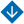No. 1, 2018
•About Advisory & Editorial Boards Aims & Scope Publication Ethics Review Process Archive Contact Information

#No. 1, 2018

Surveys

Abstract. Various concepts are presented of promising tokamaks being the leaders in solving the controlled thermonuclear fusion problem. The evolution of tokamaks is considered from ones round in vertical cross-section with a large aspect ratio up to tokamaks with a small aspect ratio including spherical ones. The classification of modern tokamaks is given. Attention is focused on the methods of plasma diagnostics by magnetic measurements outside plasma, actuators for both plasma magnetic and kinetic control, as well as for its additional heating. Plasma magnetic and kinetic models, instabilities, and disruptions are considered.

Keywords: controlled thermonuclear fusion, thermonuclear power station, tokamak, plasma, tokamak classification, diagnostics, additional heating, actuators, plasma equilibrium reconstruction, plasma magnetic and kinetic models, plasma instabilities, disruptions.
Abstract. The survey is given of some results related to approximating the functions of one and of multiple real variables. Several theorems are given of one of the classical approaches – approximating the functions by algebraic polynomials. First results on functions approximating by neural networks and fuzzy systems appeared as an answer to the significant for practical tasks question on possibility of approximating continuous functions in such ways. Later, these fields developed the same way the theory of functions approximating by algebraic polynomials did. Some results are presented, related to functions approximating by neural networks and fuzzy systems.

Keywords: approximation of functions, algebraic polynomials, neural networks, fuzzy systems.

Mathematical Problems of Control

Abstract. Methods are considered of solving fuzzy boundary value problems for second-order differential equations. Part 1 presents the fuzzy Laplace transform and fuzzy variational-iterative method for fuzzy mathematical modeling tasks solving, part 2 – fuzzy initial method and fuzzy Galerkin method of fuzzy control problems optimization. Methods listed are demonstrated on examples. In particular, the fuzzy problems are solved of fuzzy integral functional unconditional extremum and of periodic modes calculation in a fuzzy search system of automatic optimization with the extremum storing. Сonclusion the findings are given and some relevant fuzzy tasks are listed of gyroscopy, of fuzzy aircraft control of aerodynamic and space craft, which can be solved with the methods considered.

Keywords: fuzzy boundary value problem, differential equation, fuzzy Laplace transform, fuzzy variation and iterative methods, fuzzy initial method, fuzzy Galerkin method.

Analysis and Design of Control Systems

Abstract. The synthesis method is considered of non-linear object control with quadratic quality functional, based on the technique of “extended linearization” of initial mathematic model of the object. Non-linear controller parameters are determined by the solution of matrix equation of Riccati type with parameters dependent on the object state. Noted is that the main problem of such controller realization is the difficulty of finding the solution of this equation at a same speed as object operate. The decision method is suggested, based on finding the controller parameters for each time step of a control interval. The developed method of synthesis and realization of a non-linear object control is suggested to be tested by building the strategy of drug injection while cancer treatment with the use of the mathematical model of cancerous tissue growth dynamics and its interaction with normal and immune cells. The results are given of mathematical modeling, conducted to test the effectiveness of the solutions obtained.

Keywords: nonlinear differential equations, quadratic functional quality, state-dependent Riccati equation.

Control in Social and Economic Systems

Belov M.V., Novikov D.A. Network Active Systems: Planning and Stimulation Models (pp. 47-57)
Abstract. Models of network active systems including a finite set of agents interacting within a given network, characterized by the technology of their activity (the dependence of the results of their activity on their own actions and the results of the activity of other agents) are introduced and examined. The tasks of stimulation and planning are set and solved. The possibilities of using network active systems, for modeling and optimizing the activities of extended enterprises are discussed.

Keywords: active system, complex activity, extended enterprise.
Abstract. The methods are presented of fuzzy assessment of factors while analyzing the hierarchical cognitive map, describing the complex multifactor situation. The assessments of current values of factors, connected with indicators, are determined by interpreting the indicators values. The procedure of automatic design of membership functions is suggested, the peculiarity of which consists in taking into account the outliers in sampling and the differences in external conditions for regions. The assessments of factors changes are determined by interpreting the incremental values of indicators and the trends characteristics. The application of the suggested methods of factors assessment is illustrated on an example of heat energy consumption in the regions in the Russian Federation.

Keywords: hierarchical hybrid cognitive map, fuzzy assessment, energy consumption in regions.
Abstract. The algorithms are presented of information diffusion modeling and of estimating the probability of influence during marketing activities in social network, based on the independent cascade model. The calculation results are given of the probability of influence for randomly generated network with the specified characteristics.

Keywords: social networks, marketing activities, cascade model, information diffusion.